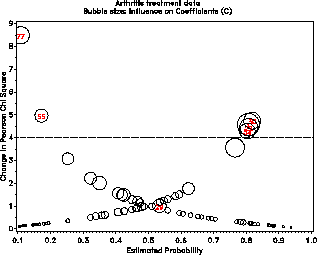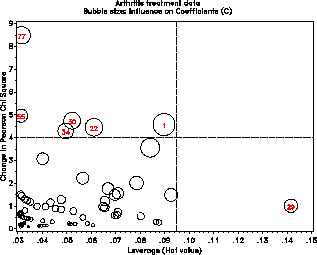inflogis Influence plots for logistic regression inflogis

# SAS Macro Programs: inflogis

\$Version: 1.4-1 (08 Jan 2012 15:44:00)
Michael Friendly
York University

## The inflogis macro (get inflogis.sas)

### Influence plots for logistic regression

The INFLOGIS macro produces influence plots for a logistic regression model. The plot shows a measure of badness of fit for a given case (DIFDEV or DIFCHISQ) vs. the fitted probability (PRED) or leverage (HAT), using an influence measure (C or CBAR) as the size of a bubble.

### Method

The macro fits the logistic model and obtains an output data set containing the diagnostic quantities which are plotted.

## Usage

inflogis is a macro program. Values must be supplied for the Y= and X= parameters.

The arguments may be listed within parentheses in any order, separated by commas. For example:

```  %include data(arthrit);
%inflogis(data=arthrit,
y=better,
x=_sex_ _treat_ age,
id=case,
);```

### Parameters

DATA=

Specifies the name of the input data set to be analyzed. [Default: `DATA=_LAST_`]

Y=

Name of the response variable

TRIALS=

Name of trials variable (for event/trials syntax)

X=

Names of predictors

CLASS=

Names of class variables among predictors (V8)

ID=

Name of observation ID variable (char)

OUT=

Name of the output data set [Default: `OUT=_DIAG_`]

GY=

Ordinate for plot: DIFDEV or DIFCHISQ [Default: `GY=DIFDEV`]

GX=

Abscissa for plot: PRED or HAT [Default: `GX=PRED`]

BUBBLE=

Bubble proportional to: C or CBAR [Default: `BUBBLE=C`]

LABEL=

Points to label: ALL, NONE, or INFL [Default: `LABEL=INFL`]

DEV=

DIFDEV/DIFCHISQ criterion for infl pts [Default: `DEV=4`]

INFL=

Specifies the criterion used to determine which observations are influential (when used with `LABEL=INFL`). [Default: INFL=%STR(DIFCHISQ > &DEV OR &BUBBLE > 1)]

LSIZE=

Observation label size. The height of other text is controlled by the `HTEXT=` goption. [Default: `LSIZE=1.5`]

LCOLOR=

Observation label color [Default: `LCOLOR=BLACK`]

LPOS=

Observation label position [Default: `LPOS=5`]

LFONT=

Font used for observation labels.

BSIZE=

Bubble size scale factor [Default: `BSIZE=10`]

BSCALE=

Bubble size proportional to AREA or RADIUS [Default: `BSCALE=AREA`]

BCOLOR=

Bubble color [Default: `BCOLOR=BLACK`]

BFILL=

Bubble fill? Options are `BFILL=SOLID `| GRADIENT, where the latter uses a gradient version of BCOLOR

REFCOL=

Color of reference lines [Default: `REFCOL=BLACK`]

REFLIN=

Line style for reference lines; 0->NONE [Default: `REFLIN=33`]

LOPTIONS=

Options for PROC LOGISTIC [Default: `LOPTIONS=NOPRINT`]

NAME=

Name of the graph in the graphic catalog [Default: `NAME=INFLOGIS`]

GOUT=

Name of the graphics catalog

### Example

The example below displays an influence plot for a logistic regression model predicting outcome of a treatment for arthritis pain, based on Sex, Treatment, and Age.

The plots display the Chi-square difference when the observation is deleted vs. both thepredicted probability of succesful outcome and the leverage with the bubble size proportional to the Cook's D measure of influence.

```%include macros(inflogis);        *-- or include in an autocall library;
%include data(arthrit);

%inflogis(data=arthrit,
y=better,
x=_sex_ _treat_ age,
id=case,
gy=DIFCHISQ,
gx=PRED HAT,
lcolor=RED, bsize=14
);
```### See also

dummy Construct dummy variables for regression models
halfnorm Half normal plot for generalized linear models
inflplot Influence plot for regression models
inflglim Influence plots for generalized linear models